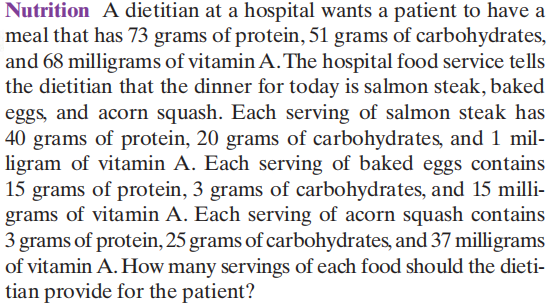### ¿Todavía tienes preguntas de matemáticas?

Pregunte a nuestros tutores expertos
Algebra
PreguntaA dietitian at a hospital wants a patient to have a meal that has $$73$$ grams of protein, $$51$$ grams of carbohydrates, and $$68$$ milligrams of vitamin A. The hospital food service tells the dietitian that the dinner for today is salmon steak, baked eggs, and acorn squash. Each serving of salmon steak has

$$40$$ grams of protein, $$20$$ grams of carbohydrates, and $$1$$ mil- ligram of vitamin A. Each serving of baked eggs contains $$15$$ grams of protein, $$3$$ grams of carbohydrates, and $$15$$ milli- grams of vitamin A. Each serving of acorn squash contains $$3$$ grams of protein, $$25$$ grams of carbohydrates, and $$37$$ milligrams of vitamin A. How many servings of each food should the dieti- tian provide for the patient?